STAT 330: 98_1

Assignment 4 Solutions

1. Chapter 6 Q 10:

1. Recall the facts: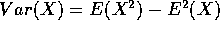,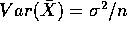and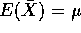Then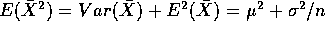which is more than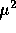so that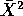is not an unbiased estimate of2. On the other hand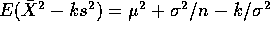which isif k=1/n.

2. Chapter 6 Q 14:

1. 525-202+1 = 324.
2. This estimate will be right if we have seen the plane with the largest number and that with the smallest number. Otherwise our estimate is an underestimate. Thus the average value of our estimator (or its expected value) must be smaller than its largest possible value which is the true number of planes, that is, the parameter value. In other words this estimate (which happens to be the maximum likelihood estimate) is very biased.

3. Chapter 6 Q 16:

1.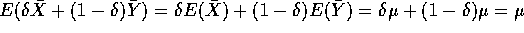so that this estimate is unbiased for any.
2. Var(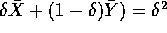Var(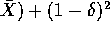Var(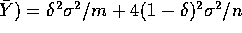. The derivative of this with respect tois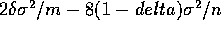which is 0 when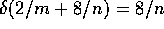or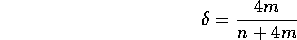3. Chapter 6 Q 22: The first population moment, the mean, is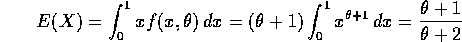This is equal to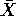when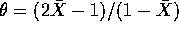or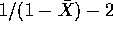. In this case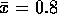and we get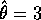.

4. The likelihood is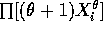and the log likelihood is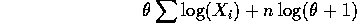The derivative of this with respect tois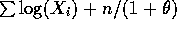which is 0 when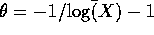which gives the estimate ?. (I haven't worked it out yet.)

4. Chapter 6 Q 32:

1. The event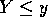is the same as the intersection of the events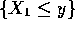,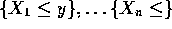. Since these events are independent we have, for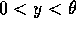,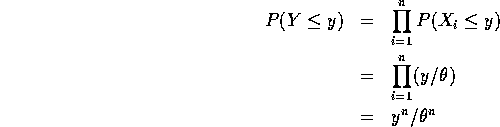The derivative of this with respect to y is the density of Y so the density is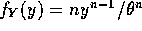which is part a).

2. We are asked to calculate the mean of Y which is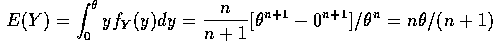which is not. Thus Y is biased but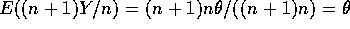so that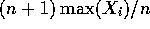is unbiased.

5. Chapter 6 Q 34:

1. When the population distribution is normal we will see that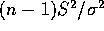has a chi-squared distribution with n-1 degrees of freedom, which is a Gamma distribution with shape (n-1)/2 and scale 2. This permits us to prove the hint since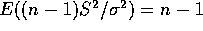and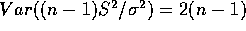. It follows that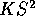has mean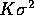, bias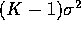and variance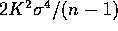. Thus the mean squared error ofis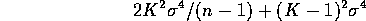which is a minimum when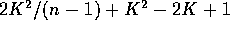is minimized. Take the derivative with respect to K and set it equal to 0 to get 4K/(n-1)+2K = 2 whose solution is K=(n-1)/(n+1).

6. Chapter 6 Q 38:

1. Here we have to multiply together the densities of all the X's and all the Y's. If we take logarithms we get the following log likelihood: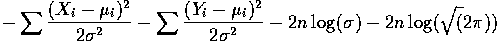The derivative with respect to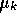is simply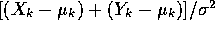which is 0 when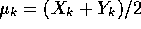. Put this in for eachand note that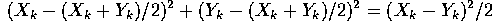Now take the derivative with respect to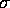to get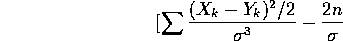which is 0 when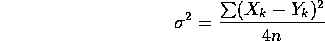2. The expected value of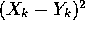is just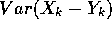because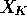and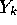have the same mean. But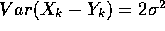so that the expected value of the mle is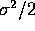. An unbiased estimate is obtained by multiplying by 2 to get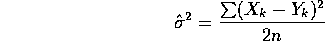Richard Lockhart
Fri Feb 6 22:47:52 PST 1998